Rg-Calculator for Platonic Solids (SAS)

Enter either the edge length (a), circum-radius (a), in-sphere-radius (a) or mid-radius (a) of a Tetrahedron / Hexahedron / Octahedron / Dodecahedron / Icosahedron. Their radius of gyration (Rg) of the solid, of the surface (faces) and of the perimeter (edges) will be calculated together with other parameters, like the volume, the surface (sum of all face areas), the perimeter (sum of all edge lengths), as well as for comparison also for a sphere with radius (=a). Units are arbitrary.

 Parameter Tetrahedron Hexahedron Octahedron Dodecahedron Icosahedron Sphere Shape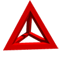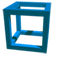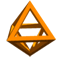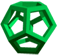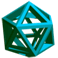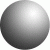edge-length a in-sphere r mid-sphere r circum-sphere r Perimeter Surface Volume Rg (edges) Rg (surface) Rg (solid)